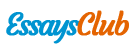# The Time Value of Money

Autor:   •  February 16, 2018  •  2,206 Words (9 Pages)  •  443 Views

Page 1 of 9

...

1 year = 4 quarters

1 quarter = 3 months

1 month = 4 weeks

1 month = 30 days

Note that the above calendar definition of time period is a standard. It is specified because there is a discrepancy in these definitions.

For example, If month = 4 weeks and year = 12 months, then ideally there should be 48 weeks in a year. But, in computations these things are usually not taken into account unless the problem asks you to specifically account for that.

Unless otherwise stated, the assumption is it is using an ordinary annuity that is a series of equal payments made at the end of consecutive periods over a fixed length of time and not annuity due that is a repeating payment that is made at the beginning of each period.

Timelines and Notation

When cash flows occur at different points in time, it is easier to deal with them using a timeline. A timeline shows the timing and the amount of each cash flow in cash flow stream. Thus, a cash flow stream of P10,000 at the end of each of the next five years can be depicted on a timeline like the one shown below.

[pic 1]

As shown above, 0 refers to the present time. A cash flow that occurs at time 0 is already in present value terms and hence does not require any adjustment for time value of money. You must distinguish between a period of time and a point of time1. Period 1 which is the first year is the portion of timeline between point 0 and point 1. The cash flow occurring at point 1 is the cash flow that occurs at the end of period 1. Finally, the discount rate, which is 12 per cent in our example, is specified for each period on the timeline and it may differ from period to period. If the cash flow occurs at the beginning, rather than the end of each year, the timeline would be as shown in Part B. Note that a cash flow occurring at the end of the year 1 is equivalent to a cash flow occurring at the beginning of year 2. Cash flows can be positive or negative. A positive cash flow is called a cash inflow; and a negative cash flow, a cash outflow.

Valuation Concepts

The time value of money establishes that there is a preference of having money at present than a future point of time. It means

- That a person will have to pay in future more, for a peso received today. For example: Suppose your father gave you P100 on your tenth birthday. You deposited this amount in a bank at 10% rate of interest for one year. How much future sum would you receive after one year? You would receive P110.

Future sum = Principal + Interest = 100+0.10×100 = P110

What would be the future sum if you deposited P100 for two years? You would now receive interest on interest earned after one year.

Future sum = 100 × 1.102 = P121. We express this procedure of calculating as Compound Value or Future Value of a sum.

- A person may accept less today, for a peso to be received in the future. Thus, the inverse of compounding process is termed as discounting. Here we can find the value of future cash flow as on today.

Techniques Of Time Value Of Money

There are two techniques for adjusting time value of money. They are:

- Compounding Techniques/Future Value Techniques

- Discounting/Present Value Techniques

The value of money at a future date with a given interest rate is called future value. Similarly, the worth of money today that is receivable or payable at a future date is called Present Value.

Future Value (Compounding)

[pic 2]

What is something worth in the future at a rate of interest. Ex. P1,000 today - put in bank for 1 year at 5%.

PV1 = P1,000 FV = PV + i

= P1,000 + P50 (P1,000 * .05 = P50)

= P1,050

PV2 = P1,050 + i

= P52.50 (P1,050 * .05 = P52.50)

= P1,102.50

Future Value of A Single Amount (Lumpsum)

The formula for calculating the Future Value of a single amount is as follows:

FVn = PV(1+r)n

Multiple Compounding Periods

Interest can be compounded monthly, quarterly and half-yearly. If compounding is quarterly, annual interest rate is to be divided by 4 and the number of years is to be multiplied by 4. Similarly, if monthly compounding is to be made, annual interest rate is to be divided by 12 and number of years is to be multiplied by 12.

The formula to calculate the compound value is [pic 3]

where, FVn = Future value after ‘n’ years

PV = Cash flow today

r = Interest rate per annum

m = Number of times compounding is done during a year

n = Number of years for which compounding is done.

Future Value of Multiple Cash Flows

The transactions in real life are not limited to one. An investor investing money in installments may wish to know the value of his savings after ‘n’ years. The formula is [pic 4]

where, FVn = Future value after ‘n’ years

PV = Present value of money today

r = Interest rate

m = Number of times compounding is done in a year.

Effective Rate of Interest in Case of Multi-Period Compounding

Effective interest rate brings all the different bases of compounding such as yearly, half-yearly, quarterly, and monthly on a single platform for

...